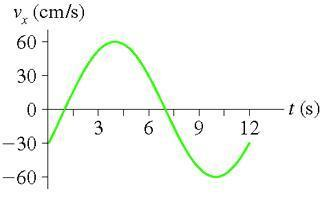## Homework Statement

What is the phase constant?The picture didn't come yet but here's a description:
It is a velocity vs. time graph. It is sinusoidal with a period of 12 s and a max velocity of 60 cm/s. The inital value for velocity at t=0 is -30 cm/s and the value for velocity at t=12 is -30 cm/s

## The Attempt at a Solution

First I found A vmax= 2pi*A/T
60= 2pi*A/12 A=114.6

ok so I tried vo= -omega*A sin(phase constant)
omega= 2pi/T 2 pi/ 12 = pi/6
-30 cm/s = -(pi/6)*114.6 sin(phase constant)

I found phase constant = .5235 rad, but that's not right

I know that there is another angle that has the sine value, but how do I know which one is the phase constant

I am really having trouble with the phase constant

Could someone help me out?

Your amplitude, A, corresponds to the maximum velocity, which in this case is 60cm/s. Your period is 12s, so by using T = 2*pi/w, in which w is angular frequency, you could find the value for w. Since you know the amplitude and angular frequency, and you know that the general form for the velocity is v = -A*sin(wt + phase constant), all you have to do is plug in numbers. At t = 0, v = -30cm/s, and since you already know the amplitude and angular frequency, you could solve for the phase constant.

Right, I think that's what I did up above, but I got the answer wrong according to mastering physics. I've checked it several times, and I don't know why it's wrong.

-30 cm/s = -(pi/6)*114.6 sin(phase constant)
.49996= sin(phaseconstant)
arc sin (.49996) = .52355 rad or 2.618 rad I don't know which one is right because at both position the particle would be moving to the left ( have a negative velocity initially like in the graph) This is what is confusing me.

Redbelly98
Staff Emeritus
Homework Helper
The amplitude is 60 cm/s.

oh, so for the velocity formula, you have to have A in cm/s...ok I see

so .955=sin(phase constant)

phase constant = 1.27 rad ?

Dick
Homework Helper
v=60*sin(wt+phase). At t=0, v=-30. Put that in. -30=60*sin(phase). sin(phase)=(-1/2). Isn't that what you want?

My book says the formula is v= -omega* Asin(omega * t + phaseconstant)

Redbelly98
Staff Emeritus
Homework Helper
Your book's formula is using the amplitude of the displacement. For this question we are using the amplitude of the quantity in the graph you showed us. That would be the velocity amplitude, 60 cm/s.

Displacement, velocity, and acceleration each have their own amplitude. We need to be clear on which one we are using.

so if I used that formula in my book, then shouldn't I come up with right answer as long as I use the amplitude from vmax=omega*A, where A is in cm. I chose to use the amplitude for displacement, so shouldn't that yield the same answer? I'm really confused about this.

Dick
Homework Helper
Yes, you are confused. If the amplitude for displacement is A, then yes, the amplitude for velocity is omega*A. But omega*A=60 from your graph. Since it's a graph of velocity. So A=60/omega. Hence the amplitude for velocity is omega*A which is omega*(60/omega). Which is 60. Which is where we started. This is making my head hurt. Can you make it stop?

I'm sorry...
Ok, so I was doing exactly what you are telling me aways up above until I got confused about the amplitude.

I wrote: .49996= sin(phaseconstant)

but the problem I was having was what value of the angle is the phase constant since arcsin .49996 gives two angles, both of which would be moving left like indicated in the graph.

Dick